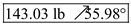# A 160-lb force P is applied at point A of a structural member. Replace P with (a) an equivalent force-couple system at C, (b) an equivalent system consisting of a vertical force at B and a second force at D.

Question-AnswerCategory: Engineering MechanicsA 160-lb force P is applied at point A of a structural member. Replace P with (a) an equivalent force-couple system at C, (b) an equivalent system consisting of a vertical force at B and a second force at D.

A 160-lb force P is applied at point A of a structural member. Replace P with (a) an equivalent force-couple system at C, (b) an equivalent system consisting of a vertical force at B and a second force at D.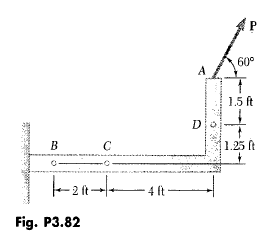Step: 1

(a)
Draw the figure to represent the force acting on the structural member.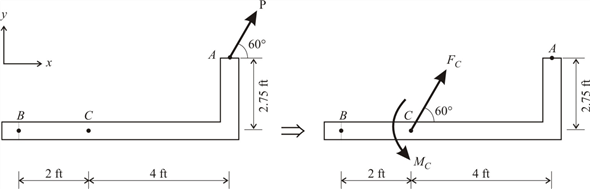Step: 2

Draw the equivalent force-couple system at C.Step: 3

Let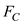andare the equivalent force and couple system at C.
The magnitude of the equivalent force at C is:Substitute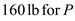in the above equation and obtain the magnitude of the equivalent force at C.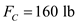And the direction of the equivalent force is same as that of the force at A.
Therefore, the equivalent force at C isStep: 4

Determine the equivalent couple at C.Therefore, the equivalent couple isStep: 5

(b)
Draw the equivalent force system consisting of a vertical force at B and a force at D.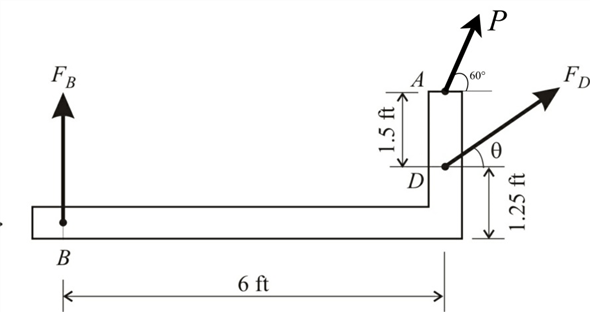Step: 6

Assume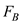to be the vertical force at B and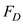to be the inclined force at D.
The forceacts at an anglefrom positive x – axis
Take the moments about D as followsSubstitutein the above equation and determine the vertical force as belowTherefore, the vertical force at B is.

Step: 7

From equilibrium condition, sum of the forces in y – direction is as follows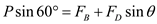Substituteandin the above equation…… (1)
From equilibrium condition, sum of the forces in x – direction is as follows:Substitutein the equation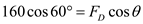…… (2)

Step: 8

Square and add the equations (1) and (2) to obtain the force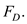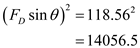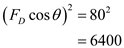Step: 9

Consider the equation (1) obtain the value of force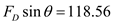Substitute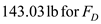.Therefore, the force at D is